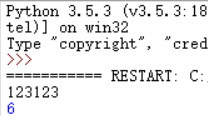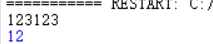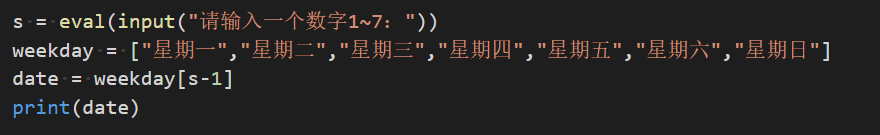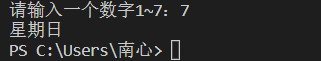• ## python输入数字求和

万次阅读 2020-01-17 10:50:23
问题 获得用户输入的一个整数N，输出N中所出现不同数字的和。...例如：用户输入 123123，其中所出现的不同数字为：1、2、3，这几个数字和为6。 解答 N = input() ss = set(N) s = 0 for i in...
问题
获得用户输入的一个整数N，输出N中所出现不同数字的和。‪‬‪‬‪‬‪‬‪‬‮‬‪‬‮‬‪‬‪‬‪‬‪‬‪‬‮‬‪‬‪‬‪‬‪‬‪‬‪‬‪‬‮‬‫‬‪‬‪‬‪‬‪‬‪‬‪‬‮‬‫‬‫‬‪‬‪‬‪‬‪‬‪‬‮‬‫‬‫‬
例如：用户输入 123123，其中所出现的不同数字为：1、2、3，这几个数字和为6。
解答
N = input()
ss = set(N)
s = 0
for i in ss:
s += eval(i)
print(s)
结果及分析可以使用set()将字符串变成集合类型，然后因为集合内的元素不能重复，所以实现去重。
举一反三
获得用户输入的一个整数N，输出N中所有数字的和。‪‬‪‬‪‬‪‬‪‬‮‬‪‬‮‬‪‬‪‬‪‬‪‬‪‬‮‬‪‬‪‬‪‬‪‬‪‬‪‬‪‬‮‬‫‬‪‬‪‬‪‬‪‬‪‬‪‬‮‬‫‬‫‬‪‬‪‬‪‬‪‬‪‬‮‬‫‬‫‬
例如：用户输入 123123，这几个数字和为12。
N = input()
lt = list(N)
r = 0
for i in lt:
r += eval(i)
print(r)可以使用list()将字符串变成列表类型，然后依次遍历，实现求和。
展开全文• Python 输入数字，输出数字对应的星期几 例如：输入数字“3”，返回“星期三” 代码： 运行结果：
Python 输入数字，输出数字对应的星期几 例如：输入数字“3”，返回“星期三” 代码：运行结果：展开全文• 输入：inList为不等长的二维数组，val为需填充的值 输出：np.array的形式输出填补完的二维矩阵 ''' maxCols = 0 #获得最大长度的子序列 for i in range( len( inList ) ): lenI = len( inList[i] ) if lenI ...
import numpy as np

def get2DlistData():
res = []

inputLine = input()  #以字符串的形式读入一行
#如果不为空字符串作后续读入
while inputLine != '':
listLine = inputLine.split(' ')  #以空格划分就是序列的形式了
listLine = [int(e) for e in listLine ] #将序列里的数由字符串变为int类型
res.append( listLine )

inputLine=input()#关键 保持循环

return res

def get2DMatData(inList,val):
'''
输入：inList为不等长的二维数组，val为需填充的值
输出：np.array的形式输出填补完的二维矩阵
'''
maxCols = 0
#获得最大长度的子序列
for i in range( len( inList ) ):
lenI = len( inList[i] )
if lenI > maxCols:
maxCols= lenI
#对每个子序列作填充
for i in range( len( inList ) ):
num = len( inList[i] )
while(num < maxCols):
inList[i].append( val )
num += 1

return np.array(inList , dtype = np.int64 )

if __name__=='__main__':
inData = get2DlistData()
print (inData)
print ('\n')

matArray = get2DMatData(inData, 0)

print( matArray )   

123 23 12
12 23 1
21
[[123, 23, 12], [12, 23, 1], ]
[[123  23  12]  [ 12  23   1]  [ 21   0   0]]
展开全文• 给用户三次猜测机会，如果三次之内猜测对了，则显示猜测正确，退出循环，如果三次之内没有猜测正确，则自动退出...lxs=int(input(“请输入一个理想数字(大于0，且为整数)：”)) num=0 while lxs&lt;=0: lxs=i...
给用户三次猜测机会，如果三次之内猜测对了，则显示猜测正确，并退出循环如果三次之内没有猜测正确，则自动退出循环，并显示‘太笨了你…’。 每个用户有 三次输错机会 且每次输错误时显示剩余错误次数 使用while循环实现， 我的方法（python）
lxs=int(input("请输入一个理想数字(大于0，且为整数)："))
num=0
while lxs<=0:
lxs=int(input("输入数字异常，请再试一次："))
continue
while lxs>0:
if num == 3:
print("太笨了你...")
break
sz = int(input("请您输入猜测的数值："))
if sz==lxs:
print("恭喜你答对了！")
break
else:
print(f"猜错了呢，再来一次吧,共有3次机会,还是{2-num}次机会")
num+=1

展开全文• 为True表示输入的所有字符都是数字，False表示不是数字或者不全部为数字 str.isalnum() 所有字符都是数字或者字母 str.isalpha() 所有字符都是字母 str.isdigit() 所有字符都是数字 str.islower...
• 寻找输入数字中的全数字（pandigital）。（4分） 题目内容： 如果一个n位数刚好包含了1至n中所有数字各一次则称它们是全数字（pandigital）的，例如四位数1324就是1至4全数字的。从键盘上输入一组整数，输出其中的全...
• Python 输入任意整数，打印输入数字是几位数 根据题目，这个比较简单，但有很多人会想的比较复杂，复杂在键盘输入0开始，后面接任意个零都可以，那么，比如，输入00024，这个输入是没有问题的，因为是整数，只是...字符串 算法 c++
• 要求：用python写一个要求用户输入数字，如果不是数字就一直循环要求输入，直到输入数字为止的代码 错误打开方式： while True: ten=input('Enter a number:') if type(eval(ten))==type(int): 　break 用这个...
• 题目：输入数字，逆序输出 知识点：列表 翻转 字符串组合 例如： 输入：123.4 输出：4.321 代码： s=input() ## 转换为列表 l=list(s) ## 列表翻转 l.reverse() ##将列表中的数字逐个输出，组合成字符串 s='' for ...
• 参考文章： ... http://www.runoob.com/python3/python3-inputoutput.html 输入 #!/usr/bin/python3 str = input("请输入："); print ("你输入的内容是: ", str) pytho...pytho
• # 输入数字使其反向输出 num = int(input("请输入一个数:")) i = 0 num1 = num while True: if num1 // 10 == 0: break i += 1 num1 = num1 // 10 sum = 0 while i &gt;= 0: sum = ...
• def random_number(num2): list2 = [] for number in range(num2): str2 = str(random.randint(0, 9)) list2.append(str2) b = " ".join(list2).replace(" ", "") return b random...random
• 学习python，开贴记录。 获取键盘输入值的函数：input（‘这里是提示’） count0 = input("请输入：") 用这个函数获取的内容都是字符串，如果键入的数字要进行运算，需要将其转变成数字。 count0=input("请输入：...计算
• 成绩分数等级为：100-90 A90-80 B80-70 C70-60 D60以下 F实现判断分数等级是一个很简单的数学问题，只要你输入的这个数字介于两个数字之间既可以判断出这个分数的等级。代码如下：a=eval(input())if a&gt;=...
• @方法1 #!/user/bin/python3 #_*_condig:utf-8_*_ result=list(set(input("\n请输入:\n"))) result=[int(n) for n in result] ...思路：set(集合)python内置函数,接受用户从控制台输入的数据并且进行处理 由于集合...
• 不用库，写了很久，一直出bug，到网上一搜，可以直接输入之后，eval(str)即可得到结果！ s=input("请输入要运算的数字") print("The result is{}".format(eval(s)))
• 当键入字符串8字符串时候，我们自己就可以判断了！...temp=input("请你输入一个数字，猜对了有奖，猜错了，没有关系：") guess=str(temp) while guess != '8':  temp=input("还没有猜对，继续猜猜看，不要放弃：")
• ## Python输入输出详解

万次阅读 2014-04-19 22:54:46
Python基本输入输出教程 python内置输入函数 python2输入 raw_input() python3输入 先在交互式解释器中查看input函数 input(...) input([prompt]) -> string Read a s...
• 一：我们在程序把输入数字当字符串处理import re print("我现在要写一个文件数字猜游戏数字游戏：") temp=input("请你输入一个数字，猜对了有奖，猜错了，没有关系：") guess=str(temp) while guess != '8': temp...正则表达式
• 在进行编程练习时，经常会遇到输入一串数字的情况，不像Ｃ语言和Java那样，可以直接对应输入Python对应输入的都是字符串，这时就需要进行转换，这时就可以使用Python的map函数来实现这一功能了。 # -*- coding:...编程练习
• s = input() try: if complex(s) == complex(eval(s)): print(eval(s)**2) except: print("输入有误") ...注意：不能直接使用eval()，否则，用户可以通过输入表达式（如100**2）输入数字，与要...
• python中计算输入数字的阶乘 代码如下： b=1 num=int(input()) for i in range (1,num): b=b*(i+1) print(b)
• 数字游戏要求：1.使用random()函数产生一个预设整数，让...当用户输入不是整数（如字母，浮点数等）时，程序会终止执行退出。当用户输入出错时给出“输入内容必须为整数”的提示。代码如下import random secret...
• python中，数字不是一个真正的对象类型，而是一组类似类型的分类。python不仅支持通常的数字类型(整数和浮点数)，而且能够通过常量直接创建数字以及处理数字表达式。此外，python为更高级的工作提供了很多高级...算法 hex 工具 random
• ## python实现猜数字游戏

万次阅读 多人点赞 2018-07-21 12:31:28
python实现猜数字游戏，主要用于练习for循环和if else。 需求如下： 1、随机生成一个1-100之间的数。 2、7次猜数字的机会，如果没有猜正确，游戏结束。 3、输入字母q，则退出游戏。 import random num = ......

# python输入的不是数字python 订阅